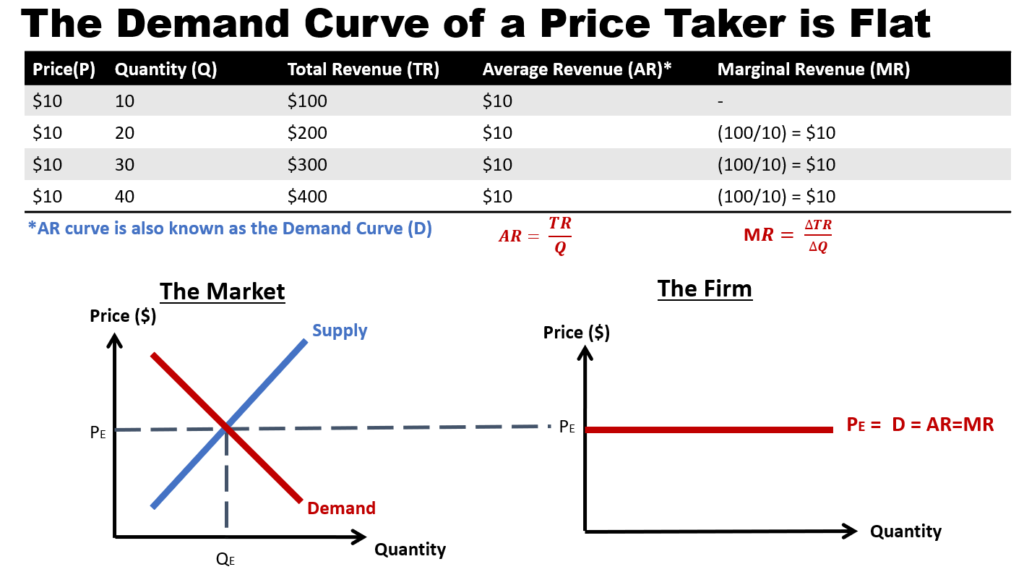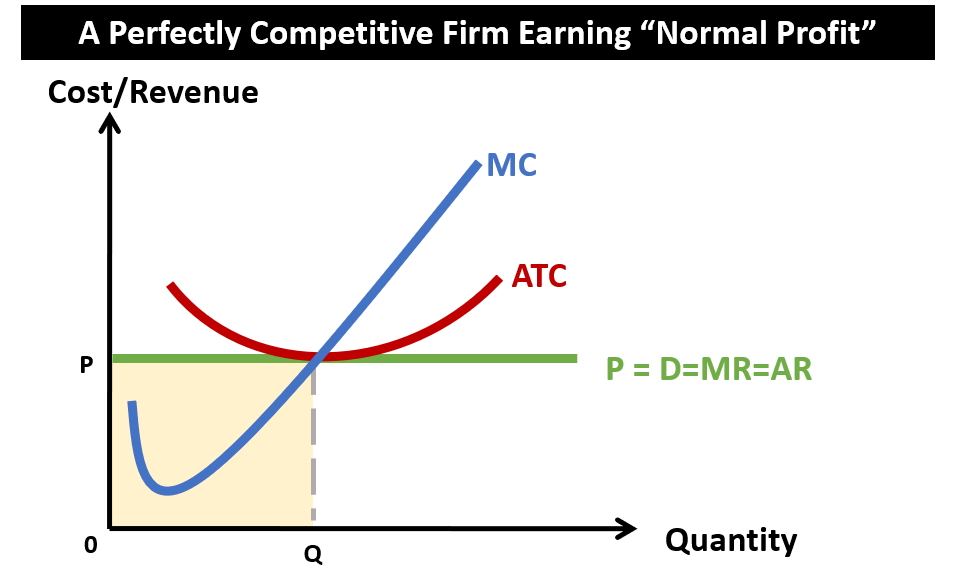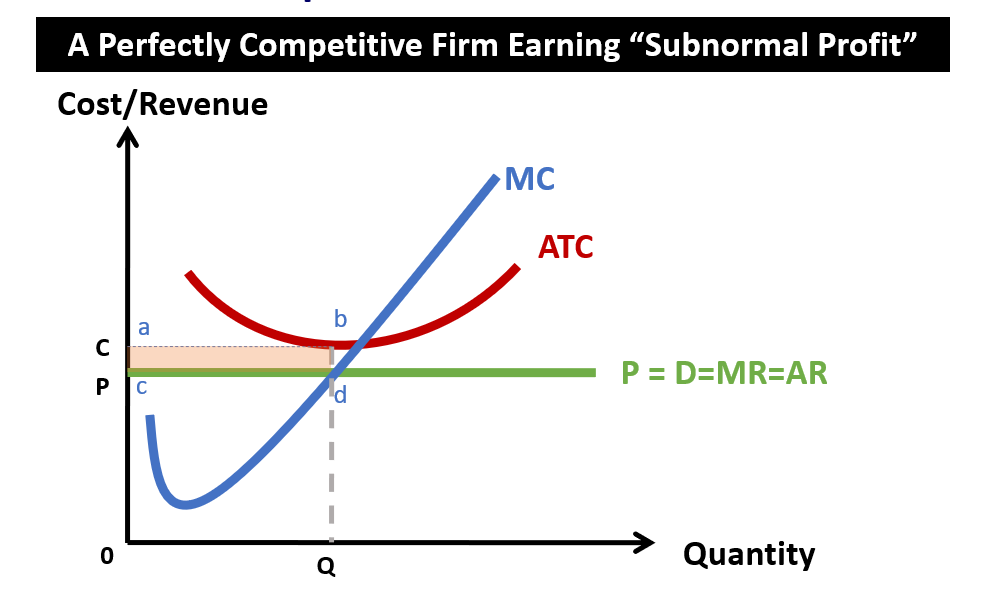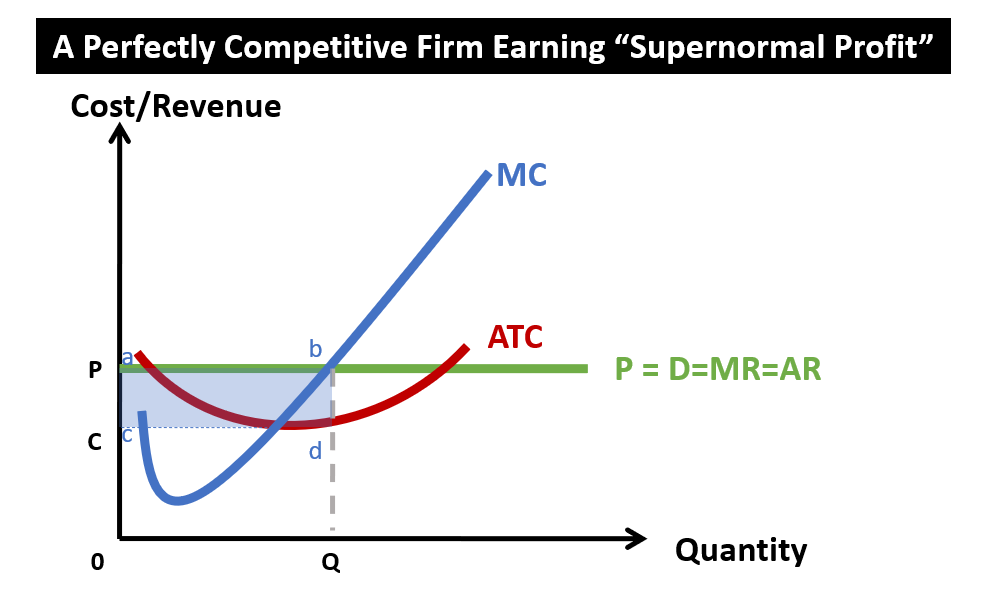# Perfect Competition

## Characteristics of Perfect Competition (PC)

### #1 Many independent producers and consumers

• Any one firm’s contribution is so small. Hence no single firm can control prices.

### #2 Standardised product

• If some firms charge higher prices, consumers would know and buy from some other firms at lower prices.
• If some firms charge lower prices, other firms would know about it and charge lower prices accordingly

### #3 No barriers to entry and exit

• If the business is profit making, it would attract more new firms to enter the market to compete.
• If the business is losing money, lost-making firms can easily exit.

### #4 Firms are "price takers"

• No one firm dominates or control the price of the market.
• They just accept the price set by the market

### #5 Perfect knowledge of market by firms and consumers

• If some firms charge higher prices, consumers would know and buy from some other firms at lower prices.
• If some firms charge lower prices, other firms would know about it and charge lower prices accordingly

### #6 Distance between firms selling homogeneous goods must be very close

• No one firm would be in a more “strategic location” than others, such that no consumers would pay more for convenience.

## Understanding the demand curve of a firm under Perfect Competition (PC)

If we follow the above-mentioned characteristics (or assumptions) of a firm under perfect competition (PC), the resulting demand curve will be flat. Why?

• Since the firm is a price-taker, it is unable to vary the price of its products. It can only accept the price set by the supply and demand in the market.
• Given that the price is fixed, the average revenue (AR) will be determined by the price (p). (Note: AR = TR/Q = P*Q/Q = P)
• If the price is fixed, then the variation in total revenue will increase in the same proportion as the quantity sold. This means that the marginal revenue (MR) will be the same as the price (P). (Note: MR = ΔTR/ΔQ = (P*ΔQ)/ΔQ = P).
• Demand curve is another name of the Average Revenue (AR) curve, since demand is simply the total revenue over the quantity sold. But since AR = MR = P, and that D = AR, therefore D=AR=MR=P.## Understanding the various profits of a firm under Perfect Competition (PC)

### #1 Normal ProfitWhen a perfectly competitive firm is earning normal profit, it means that its total revenue is equal to the total cost.

In economics, the calculation of the total cost includes the opportunity cost. Hence, when a firm is earning normal profit, it also means that its economic profit is equal to zero. At that point, the perfectly competitive firm is earning the same amount  as it would if the resources were used to produce the next best alternative.

The revenue earned is not great, but it is sufficient to compensate the firm’s owners for the time and money they have invested to just keep the business going. Therefore, this firm would not leave the market. Knowing this, other firms would also not be incentivsed to enter the market.

In the diagram, the total revenue is P*Q (the yellow area), while the total costs is also P*Q. Hence, the economic profit is zero.

### #2 Subnormal Profit

When a perfectly competitive firm is earning a subnormal profit, it means that the total cost is greater than the total revenue. Recall that the total cost includes the opportunity cost. So essentially, the perfectly competitive firm is chalking up economic losses. This loss is shown by the red rectangular area abcd.

In economics, subnormal profit in the perfect competitive market is a short-run phenomena. Eventually, some firms in the market may decide to exit the industry. With fewer firms, the market supply curve will decrease and thereby increasing the market price. This adjustment will make the losses smaller and smaller, till the economic losses eventually disappear. When that happens, the firm is back to earning only normal profit in the long run.### #3 Supernormal ProfitWhen a perfectly competitive firm is making supernormal profit, it means that the total revenue from the business is more than the total cost (even after considering the opportunity cost of the next best alternative forgone). The size of the supernormal profit is given by the blue rectangular areas abcd.

However, supernormal profit in a perfectly competitive market is a short-run phenomena. Eventually, knowledge of supernormal profit will entice entry of more firms, leading to an increase in supply of goods. This excess supply will bring down the market price, and eventually eliminating the supernormal profit. Hence, in the long run, the perfectly competitive firm can only earn normal profit.